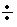Name:    2nd Grade Math Proficient Test 1

Multiple Choice
Identify the choice that best completes the statement or answers the question.

1.

Algebraic Concepts – Ordered Pairs – RIT 181 – 190Which letter is at point (5,2)?
 a. Point A d. Point D b. Point B e. Point E c. Point C

2.

Algebraic Concepts - Patterns, Sequences, Functions - RIT 181 - 190

1, 4, 7, 1 0 ____, ______.

What are the next two numbers in the pattern?
 a. 12, 14 d. 13, 17 b. 14, 15 e. 13, 16 c. 11, 12

3.

What is the missing number in this pattern?
10    8    ?    4     2
 a. 6 d. 3 b. 9 e. 7 c. 5

4.

Sally is selling candy bars for her school.  Use the table to answer the question.  How many candy bars will Saly sell on Thursday?

Day           Bars Sold
Mon.                 3
Tues.                6
Wed.                 9
Thurs.              ?
 a. 4 d. 10 b. 5 e. 8 c. 12

5.

Algebraic Concepts - Properties - RIT 181 - 190

(5 + 4) + 3 = 12                 5 + (4 + 3) = 12
9 + 3 = 12                          5 + 7 = 12

Which property does this show?
 a. Associative Property d. Addition Property b. Commutative Property e. Subtraction Property c. Zero Property

6.

Algebraic Concepts- Solving Equations and Inequities-RIT 181- 190

If 6 x B = 12,   B = ?
 a. 6 d. 5 b. 3 e. 12 c. 2

7.

5  +  _____  = 7
 a. 3 d. 2 b. 7 e. 12 c. 5

8.

12  + ____  = 14
 a. 26 d. 12 b. 1 e. 2 c. 3

9.

Algebraic Concepts – Patterns, Sequences, Functions – RIT 191 – 200

24,     28,  32,  36,  40,  _____
Which number comes next?
 a. 40 d. 48 b. 44 e. 41 c. 43

10.

Algebraic Concepts- Solving Equations and Inequities-RIT 181- 190

2,643  >  ______
Which number makes this a true statement?
 a. 2,645 d. 2,648 b. 4,342 e. 6,243 c. 2,633

11.

If _____ + 300 = 820, then ____ equals?
 a. 20 d. 520 b. 500 e. 620 c. 420

12.

If 10 x  ___  = 80, then _____ equals?
 a. 8 d. 70 b. 0 e. 800 c. 80

13.

If 42____ = 7, then ____ equals?
 a. 6 d. 49 b. 36 e. 5 c. 7

14.

33 = ______
 a. 6 d. 81 b. 27 e. 9 c. 3

15.

Evaluate 3x + 4 if x = 2
 a. 2 d. 30 b. 4 e. 36 c. 10